### Shallow water calculations

Calculations for incompressible fluids with a free surface are quite important in marine and oceanographic applications. The challenging part here is to predict the location of the free surface. A special subcategory are the problems in which the depth of the fluid is small compared to the other dimensions. In that case the general equations can be reduced to the so-called shallow water equations. These equations have a very similar behavior as the Navier-Stokes equations for compressible fluids, treated in the previous section. Linearization of these equations leads to the linear shallow water equations treated in Section 6.9.10.

Starting point for the derivation of the shallow water equations are the conservation of mass and momentum for incompressible fluids: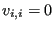(481)

and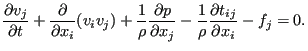(482)

Now, the depth-direction of the fluid is assumed to coincide with the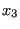-direction. The momentum equation in the-direction now reads: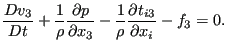(483)

The velocity in depth direction (first term) is assumed to be neglegible as well as the viscous stress components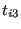. Furthermore, the volumetric force density is assumed to reduce to the gravity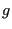. Consequently, one obtains: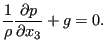(484)

Now, the depth is supposed to be composed of two contributions: a portion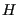extending from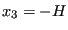(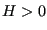) up to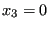, and a portion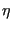extending fromup to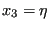(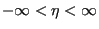), so that the depth h amounts to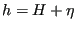(Figure ...). Integrating the above equation and applying the boundary condition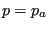for, where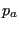is the atmospheric pressure, one obtains: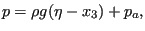(485)

expressing the the pressure increases linearly from the surface into the depth direction.

The conservation of mass equation can be integrated in z-direction as follows: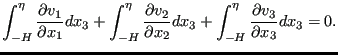(486)

Applying the Leibniz rule to the first two equations and direct integration to the last term leads to: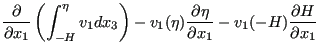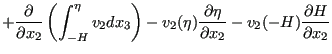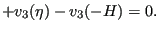(487)

The velocity at the bottom is zero, i.e.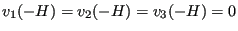. Furthermore, a mean velocity is now defined by: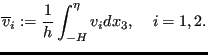(488)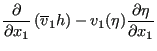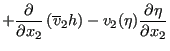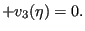(489)

Now, the vertical velocity at the free surface can be written as: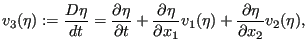(490)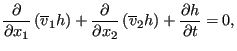(491)

since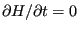. With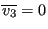one can also write: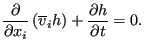(492)

This is identical to Equation 451 (conservation of mass for a compressible fluid) in which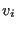is replaced by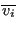and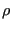by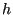.

Integrating the momentum equation, i.e. Equation (482) in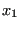and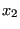direction across the depth leads to: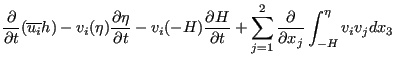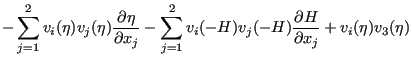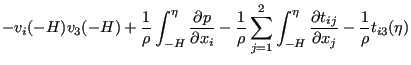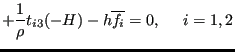(493)

Since the velocity at the bottom is zero, the third, sixth and eight term vanish. Due to the definition of the vertical velocity at the free surface, Equation (490) the second, fifth and seventh term also disappear. Due to Equation (485) the ninth term amounts to: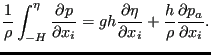(494)

The fourth term is approximated by: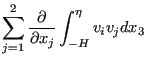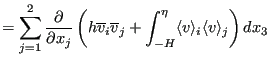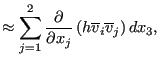(495)

and the tenth term is neglected. This leads to: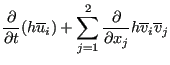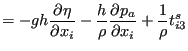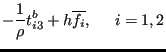(496)

Now,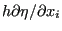can also be written as: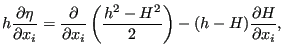(497)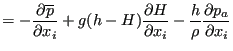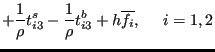(498)

were an artifical pressure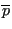has been defined by: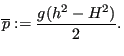(499)

Since there is no variation in depth direction and setting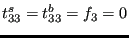this also amounts to: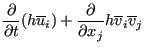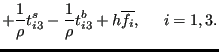(500)

This amounts to the conservation of momentum equation (447) for a compressible fluid with the densityreplaced by,replaced by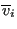,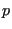replaced by,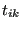neglected and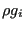replaced by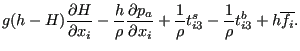(501)

The friction stress at the bottom is frequently modeled by a hydraulic resistance type formula such as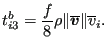(502)

The energy equation can be integrated in a similar way. I can be used of some fluid at a higher temperature is released into the flow and one would like to study the spread of the heat. The equation for incompressible flow runs: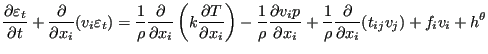(503)

Integrating from -H toyields: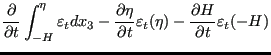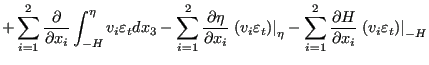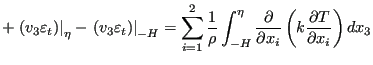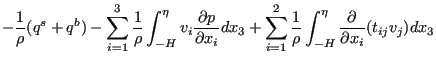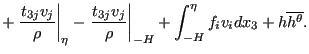(504)

Terms 3, 6 and 8 on the left hand side and term 6 on the right hand side are zero (no velocity at the bottom and no change in time of the bottom level). Terms 2, 5 and 7 on the left hand side disappear due to Equation (490). The integral in the first term on left hand side is replaced by definition by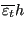and the integral in the fourth term is approximated by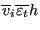. The variables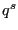and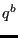in the second term on the right hand side are the heat flux flowing out of the fluid at the surface and the bottom, respecitively.

Substituting the expression for the pressure, i.e. Equation (485) into the third term on the right hand side yields (the summation in that term really only extends from 1 to 2 since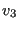is neglegible):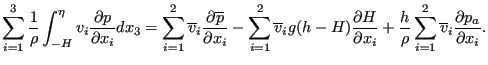(505)

The first and the fourth term on the right hand side is neglected and the eighth term is approximated by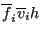. This finally yields: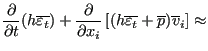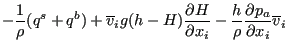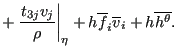(506)

This is equivalent to the energy equation for compressible fluids withreplaced byand appropriate source terms. The neglection of the stress and conduction terms (except in-direction) can be obtained by setting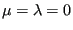. No specific gas constant has to be defined. The parameter COMPRESSIBLE on the *CFD card has to be replaced by the SHALLOW WATER parameter. The pressure initial and boundary conditions have to be replaced by conditions for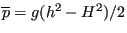. Ifcorresponds to the fluid surface in rest, the initial conditions ususally reduce to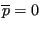.